# Time Interval with respect any specific data

I am a student and I want to create a project in in which a different persons spending time on a particular table.
Kindly help me .

Hi @Suraj_Verma, what code have you already tried? This looks like something you can probably do using vlines:

``````import matplotlib.pyplot as plt
fig, ax = plt.subplots()
_ = ax.vlines(x=[1,1,2,3], ymin=[2,5,1,3], ymax=[4, 7, 4, 5])
``````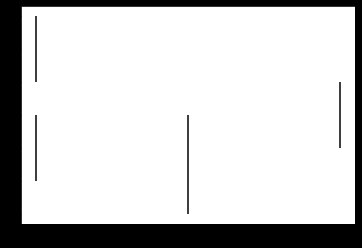where ymin is a list of bottoms of each line and ymax is a list of tops of each line.

If the suggestion made by @story645 fits your needs, I wonder if you’d need to take this one step further and color-code the lines to represent the people assigned to each table. Here’s a little something I knocked up using the code provided by @story645 as a starting point. It shows the same person (“bob”) being assigned to different tables at different times.

Hope this helps
Laurence

``````import numpy as np
import matplotlib.pyplot as plt
import matplotlib.patches as mpatches

fig, ax = plt.subplots()

# define color codes and names for each person
color = ['#BB0000', '#00BB00', '#0000BB', '#BBBB00']
name = ['rita', 'bob', 'sue', 'matt']

# plot lines
_ = ax.vlines(x=[1,1,2,3], ymin=[2,5,1,3], ymax=[4, 7, 4, 5],
colors=[color,color,color,color])

# set x axis labelling, display at an angle (60 degrees)
_ = ax.set_xticks(np.arange(5))
_ = ax.set_xticklabels(['','T-01',' T-02','T-03',''],
rotation=60)

# set y axis labelling
_ = ax.set_yticks(np.arange(10))
_ = ax.set_yticklabels(['','01:00','02:00','03:00','04:00',
'05:00','06:00','07:00','08:00',''])

# create a legend
legend = [mpatches.Patch(color=p, label=f'{name[p]}')
for p in enumerate(color)]
_ = ax.legend(handles=legend, ncol=len(legend),
bbox_to_anchor=(0, 1.02, 1, .102))

# required to fit rotated x labels
fig.tight_layout()
``````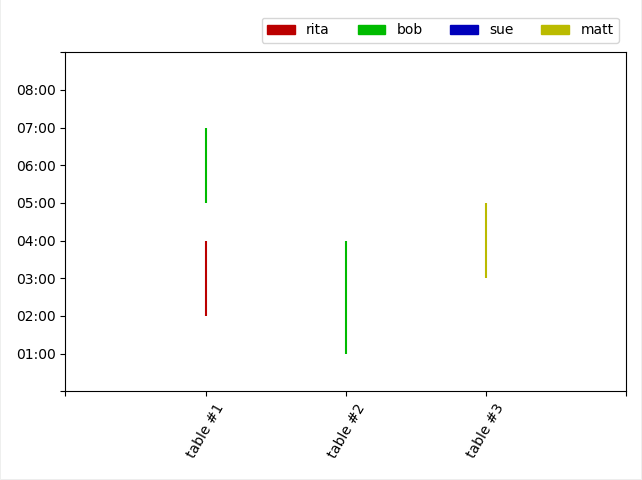`vlines` takes a label argument, so you can also loop over/plot each person individually, which might be easier in terms of keeping track:

``````fig, ax = plt.subplots()
_ = ax.vlines(x=[1,1,3,6], ymin=[2,5,1,3], ymax=[4, 7, 4, 5],
color='green', label='bob')
_ = ax.vlines(x=[2,2,4,5], ymin=[2,5,1,3], ymax=[4, 7, 4, 5],
color='blue', label='alice')
_ = ax.legend()
``````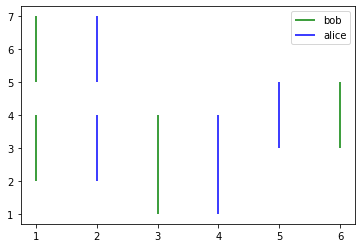1 Like

Ah yes. I like that way of structuring things. One set of vlines per person. Nice.1 Like

Thank you @story645 and I love ur effort !!

1 Like

Thank you @laurence.molloy and I love ur effort !!

1 Like

Also the time stamps can be plotted directly rather than having to relabel, I just used integers because it was easier. What format is your data in?

Yah, I was trying but relabeling was quite complex and format of my data is str.

Not a Matplotlib trick as such but, if your timings are by the hour, you can use integers (1,2,3) or integer strings (‘1’,‘2’,‘3’) to represent hours in your input data and a single line of regular expression magic to map those to formatted time strings in your y axis labels, thus

1 / ‘1’ => 01:00
2 / ‘2’ => 02:00
etc…

So, using my previous code snippet, you simply change

``````# set y axis labelling
_ = ax.set_yticks(np.arange(10))
_ = ax.set_yticklabels(['','01:00','02:00','03:00','04:00',
'05:00','06:00','07:00','08:00',''])
``````

to

``````# set y axis labelling
hours = np.arange(10)
_ = ax.set_yticks(hours)
_ = ax.set_yticklabels(re.sub('(\d+)',r'\1:00', str(i)) for i in hours)``````
1 Like

If your input data is of the format ‘02:00’ you can also do something similar prior to plotting to convert it to numerals:

``````# example data
start_times = ['02:00','05:00','01:00','03:00']
end_times = ['04:00','07:00','04:00','05:00']

# translate time strings to integer numerals
start_times = [int(re.sub('(:.*)\$','', s)) for s in start_times]
end_times = [int(re.sub('(:.*)\$','', s)) for s in end_times]

# plot lines
_ = ax.vlines(x=[1,1,2,3], ymin=start_times, ymax=end_times)``````
1 Like

Any chance you can provide the code you’re using, a small subset of the data, and what figure it’s generating? It’ll be a lot easier to provide suggestions with that information.

1 Like

I think going in reverse and using a formatter might be less work. Particularly the `FormatStrFormtter`
Something like:

``````import matplotlib.pyplot as plt
import matplotlib.ticker as mticker

fig, ax = plt.subplots()
_ = ax.vlines(x=[1,1,3,6], ymin=[2,5,1,3], ymax=[4, 7, 4, 5], color='green', label='bob')
_ = ax.vlines(x=[2,2,4,5], ymin=[2,5,1,3], ymax=[4, 7, 4, 5], color='blue', label='alice')
_ = ax.yaxis.set_major_formatter(mticker.FormatStrFormatter('%02d:00'))
_ = ax.legend()
``````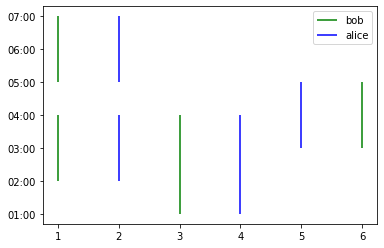2 Likes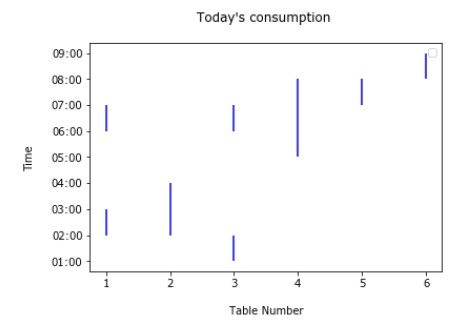Thank you So much !!!

1 LikeThank you so much !!!

1 Like

@Suraj_Verma You’re welcome. I learned one or two things myself as well.

@story645 nice solution. Matplotlib is full of surprises. I’ll need to read up on ticker formatters.2 Likes

Here’s the Python 3 format string version that I couldn’t find the first time:

``````import matplotlib.pyplot as plt
import matplotlib.ticker as mticker

fig, ax = plt.subplots()
_ = ax.vlines(x=[1,1,3,6], ymin=[2,5,1,3], ymax=[4, 7, 4, 5], color='green', label='bob')
_ = ax.vlines(x=[2,2,4,5], ymin=[2,5,1,3], ymax=[4, 7, 4, 5], color='blue', label='alice')
_ = ax.yaxis.set_major_formatter(mticker.StrMethodFormatter('{x:02.0f}:00'))

_ = ax.legend()
``````

Hey Everyone thanks for your efforts, and now I want to specify the the hours along with minutes , is it possible !

like somebody came at 06:30 but our graph shows that he/she came at 06:00.

Quick answer, as I have to head out in a few minutes, but here’s a code snippet that shows how you can throw arbitrary times into the plot. Sorry, not in @story645’s one plot per person format as I’m in a hurry.

All it needs is feint major ticker horizontal grid lines, which I can find the code for when I’m back

Hope this helps
Laurence

``````import matplotlib.dates as mdates

fig, ax = plt.subplots()

# define color codes and names for each person
color = ['#BB0000', '#00BB00', '#0000BB', '#BBBB00']
name = ['rita', 'bob', 'sue', 'matt']

ymin = ['02:30', '05:00', '01:15', '03:00']
ymax = ['04:30', '07:45', '04:15', '05:30']

ymin = pd.to_datetime(ymin, format='%H:%M')
ymax = pd.to_datetime(ymax, format='%H:%M')

myFmt = mdates.DateFormatter('%H:%M')
ax.yaxis.set_major_formatter(myFmt)

# plot lines
_ = ax.vlines(x=[1,1,2,3], ymin=ymin, ymax=ymax,
colors=[color,color,color,color])
``````

2 Likes

Try this to add feint horizontal grid lines to the plot:

ax.grid(axis=‘y’, b=True, which=‘major’, color=’#666666’, linestyle=’-’, alpha=0.25)

1 Like